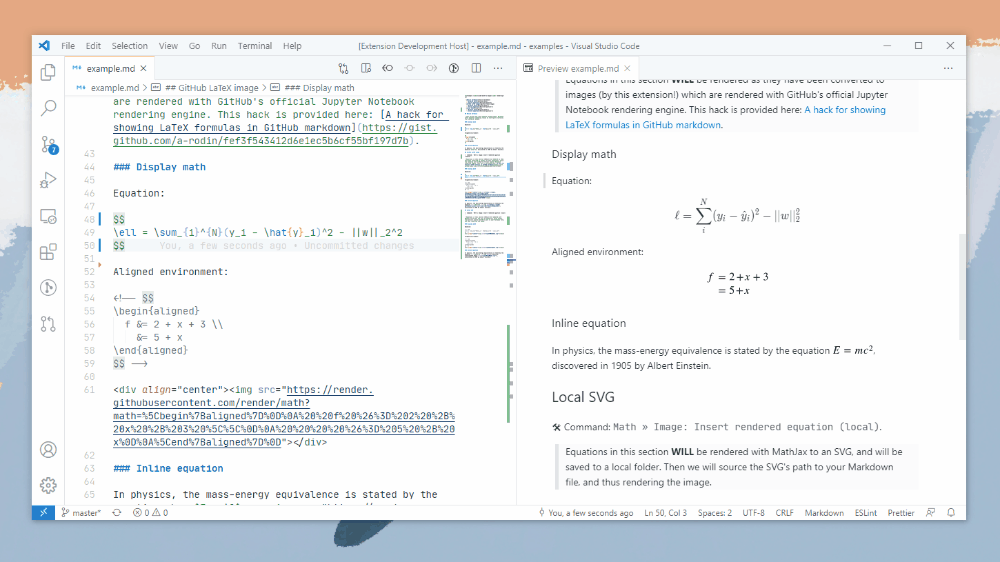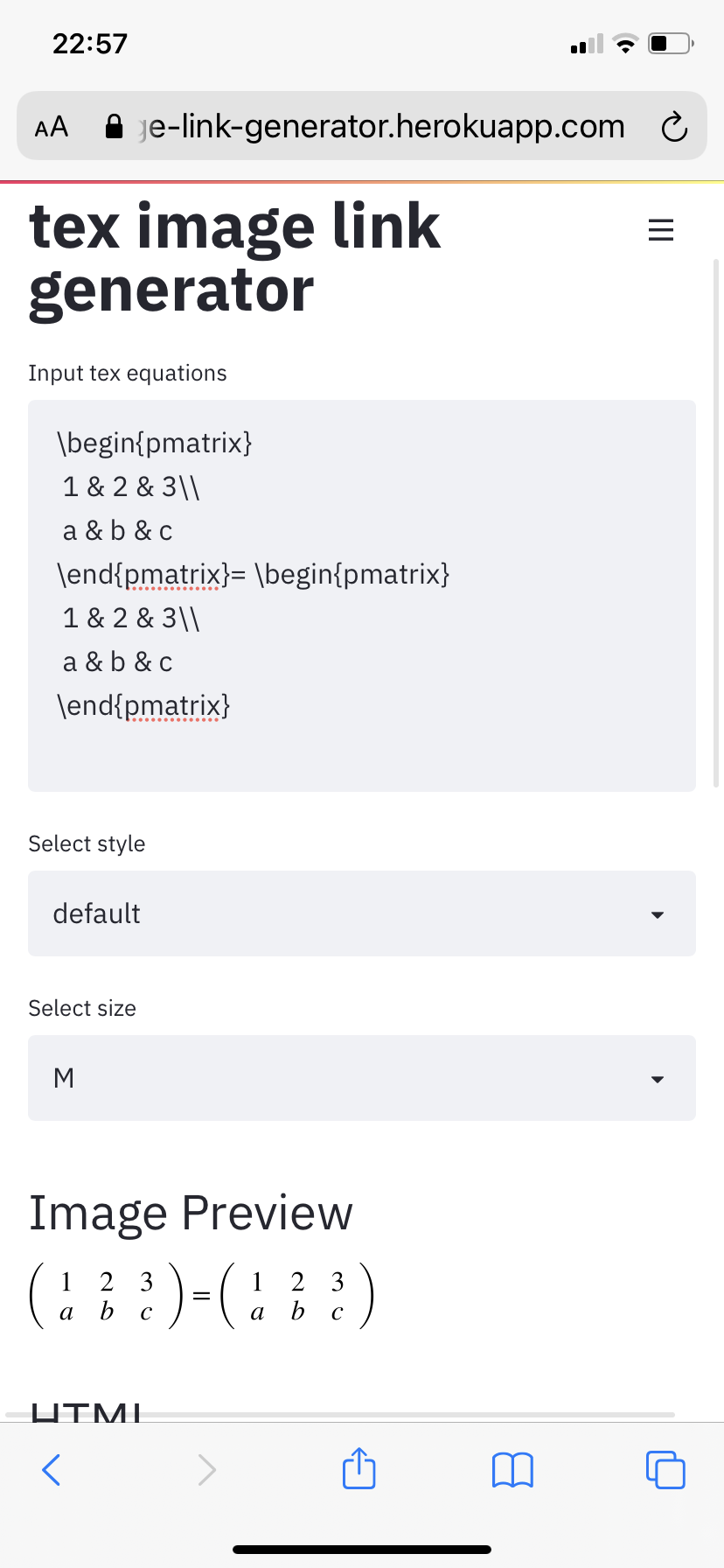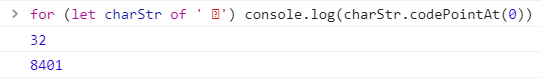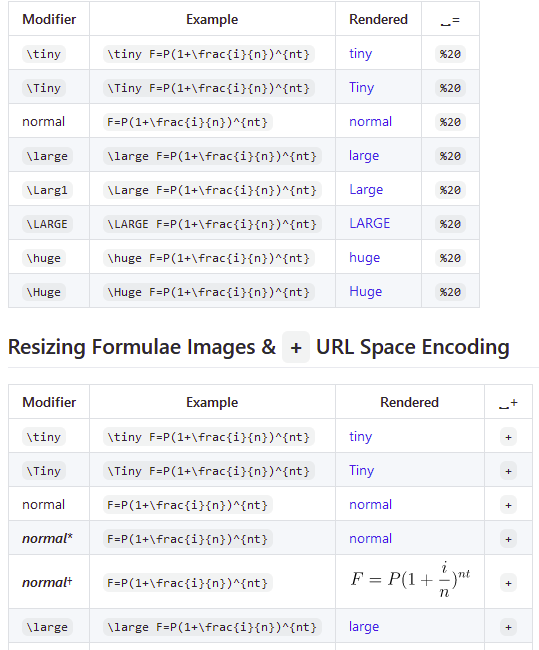Skip to content
{{ message }}

Instantly share code, notes, and snippets.

# a-rodin/A hack for showing LaTeX formulas in GitHub markdown.md

Last active Jun 11, 2021

## Problem

A lot of GitHub projects need to have pretty math formulas in READMEs, wikis or other markdown pages. The desired approach would be to just write inline LaTeX-style formulas like this:

$e^{i \pi} = -1$

Unfortunately, GitHub does not support inline formulas. The issue is tracked here.

## Investigation

However, it does support them in Jupyter notebooks, scroll below to see an example. One might question how does it work in notebooks. It turns out that instead of relying on MathJax as nbviewer does, GitHub's notebooks renderer converts inline math to images with source URLs that look like this:

https://render.githubusercontent.com/render/math?math=e%5E%7Bi%20%5Cpi%7D%20%3D%20-1&mode=inline

Inspecting the URL, it is possible to notice that:

1. The &mode=inline part is not required, the URL still returns the same image without it:

2. Modern browsers encode URLs automatically, thus this link would work as well:

## Solution

So the solution would be to insert this code in Markdown files:

<img src="https://render.githubusercontent.com/render/math?math=e^{i \pi} = -1">

and such an image would be rendered as.

This is more clumsy than just $e^{i \pi} = -1$, but it is still possible to write the formula by hand directly to the Markdown file this way.

Note that this syntax for image insertion would not work because the URL contains spaces:

![formula](https://render.githubusercontent.com/render/math?math=e^{i \pi} = -1)

## Addition from 2019-10-30

I made a small app that allows to generate Markdown code from LaTeX using the method described above automatically.

Sorry, something went wrong. Reload?
Sorry, we cannot display this file.
Sorry, this file is invalid so it cannot be displayed.

###tpenguinltg commented May 10, 2019 • edited

 The Markdown image syntax should work if you replace the spaces with %20. Testing below:###daos20x15 commented Aug 27, 2019

 I wanted to write a formula with a "+" sign, but its apparently not working, any thoughts on how to make the "+" show? 

###a-rodin commented Aug 27, 2019 • edited

 @daos20x15 + symbol is used to escape spaces in URI encoding and the formula has to be either properly escaped or at least don't conflict with possible escaped version created by the browser. The browser replaces spaces by + or %20 before sending to the server, so when it encounters + it assumes that it is already escaped and sends + sign as is, which is decoded by the server as a space. So + should be replaced by encoded version, which is %2B. To be short: a formula with pluses should be written like this   which would be displayed as. To encode other symbols or entire formulas you can use encodeURIComponent function from you browser's developer console.

###daos20x15 commented Aug 27, 2019

 @daos20x15 + symbol is used to escape spaces in URI encoding and the formula has to be either properly escaped or at least don't conflict with possible escaped version created by the browser. The browser replaces spaces by + or %20 before sending to the server, so when it encounters + it assumes that it is already escaped and sends + sign as is, which is decoded by the server as a space. So + should be replaced by encoded version, which is %2B. To be short: a formula with pluses should be written like this   which would be displayed as ![](https://render.githubusercontent.com/render/math?math=e^{i %2B\pi} =x%2B1). To encode other symbols or entire formulas you can use encodeURIComponent function from you browser's developer console. This is very useful. Thanks a lot @a-rodin

###pedrolemoz commented Oct 29, 2019 • edited

 How to use this method to render brackets and sets (from mathematic)? I tryed these: ![formula](https://render.githubusercontent.com/render/math?math=A%20-%20B%20=%20\{x%20\in%20\U%20\mid%20x%20\in%20A%20\land%20x%20\notin%20B%20\}) ![formula](https://render.githubusercontent.com/render/math?math=A,%20B%20\in%20\U,%20x%20\in%20\Z) And I get:But, if I try to add a bracket... ![formula](https://render.githubusercontent.com/render/math?math=\left%20\{%20\right%20\})And if I try to add a set notation... ![formula](https://render.githubusercontent.com/render/math?math=\N\Z\R\Q\C)These aren't sets! How can I get what I want?

###a-rodin commented Oct 30, 2019 • edited

 @pedrolemoz Braces ({}) need to be escaped by \ in LaTeX, square brackets ([]) can be used as is in LaTeX, but they collide with Markdown syntax and thus need to be URI-encoded. I made a small app that converts LaTeX to Markdown code automatically. Here is an example that uses both braces, square brackets, and sets.

###jaimet commented Nov 21, 2019

 Hi. Would you consider making a repo out of this? (I would like to add some content such as corrections and additional documentation, but I don't want to do it via comments on a gist.) Thank you for the original "hack" - it's brilliant!

###cjuicey commented Dec 11, 2019

 Worked. Thanks for the upload!

###chewxy commented Jan 17, 2020

 This is brilliant. Thanks

###Mekahou commented Feb 17, 2020

 This is awesome. Thanks a lot!

###behrouz-bakhtiari commented Feb 24, 2020

 Thank you very much. This is very useful. I am a little disappointed at Github, but this method is a life saver.

###joyjeni commented Mar 20, 2020

 %2B Thankyou It worked

###kartynnik commented Apr 4, 2020

 @a-rodin Why are you using KaTeX for previews in your app? It seems natural to give the "WYSIWYG" feedback by showing the exact image as rendered by the GitHub's renderer.

###manueltonneau commented Apr 5, 2020

 Thank you very much, very cool stuff! If anybody was wondering how to render the equation in a bigger size, just write height="50" width="500" (just adapt the values to your case) after the last " and before >.

###a-rodin commented Apr 5, 2020

 @kartynnik The preview images are returned by GitHub, so they look exactly the same as they would on GitHub. However, in case of parsing errors GitHub doesn't provide an error message, but just returns HTTP 406 error code. In such cases the formulas are additionally parsed using KaTeX and if KaTeX parser detects any error, such an error is displayed to the user to make it easier to fix it. That's why the previews are not instant, but take some time to show up.

###kartynnik commented Apr 5, 2020

 @a-rodin Makes perfect sense, thanks!

###whogopu commented May 2, 2020

 @a-rodin This is really great

###ninjakx commented May 11, 2020 • edited

 It's not working for me in .rst file Works here:There It's excluding the circular bracket.

###vrn25 commented May 23, 2020

 @a-rodin is there any way for using $$e^{i \pi} = -1$$?

###psambit9791 commented Jun 1, 2020

 Much appreciation for sharing this. Saved me a good bit of time.

###ghost commented Jun 13, 2020

 This is wonderful. Thank you for posting this!!

###jk13o3lll commented Jun 23, 2020 • edited

 Here is a simple script to convert the equation into html: https://jsfiddle.net/8ndx694g/ For the following example, it works well. \Huge \begin{align*} x&=1\\ x+y&=2\\ P&=\begin{bmatrix}p_1\\p_2\end{bmatrix} \end{align*} 

###cga203 commented Jun 23, 2020

 Hello @a-rodin, the link to your small app is broken.

###psireal9 commented Jun 24, 2020 • edited

 The ![formula][url] doesnt work in an evironment ... (for example if you want to the your equation inside or "")

###mubaraqwahab commented Jul 8, 2020

 Thank you!

###zuyazii commented Jul 30, 2020

 Here is a simple script to convert the equation into html: https://jsfiddle.net/8ndx694g/ For the following example, it works well. \Huge \begin{align*} x&=1\\ x+y&=2\\ P&=\begin{bmatrix}p_1\\p_2\end{bmatrix} \end{align*}  Thank you! It helped me a lot!

###spencerwooo commented Aug 2, 2020 • edited

 Hi guys, me and my friends made a VS Code extension to convert math equations into rendered SVGs here: GitHub- vscode-math-to-image / VS Marketplace - Math to Image. We used the method mentioned in this gist to render external SVGs with GitHub math rendering servers. We also added a feature to render them into local SVGs with MathJax. Feel free to try it out! ❤###masakiaota commented Aug 10, 2020

 https://gist.github.com/a-rodin/fef3f543412d6e1ec5b6cf55bf197d7b#addition-from-2019-10-30 I made a small app that allows to generate Markdown code from LaTeX using the method described above automatically. This link doesn't work... so I made new one https://tex-image-link-generator.herokuapp.com/

###juangburgos commented Aug 27, 2020

 Thank you @masakiaota, you are the best. Unbelievable that github does not prove a built in solution for this. Gatlab has it for years now, although they broke it recently which also sucks.

# Thanks

###hypercube-software commented Sep 23, 2020 • edited

 You may also take a look on my tool: latexMarkdown2Markdown

###Spir4L commented Sep 24, 2020

 @pedrolemoz Braces ({}) need to be escaped by \ in LaTeX, square brackets ([]) can be used as is in LaTeX, but they collide with Markdown syntax and thus need to be URI-encoded. I made a small app that converts LaTeX to Markdown code automatically. Here is an example that uses both braces, square brackets, and sets. Hey, the link is broken!

###Spir4L commented Sep 24, 2020

 https://gist.github.com/a-rodin/fef3f543412d6e1ec5b6cf55bf197d7b#addition-from-2019-10-30 I made a small app that allows to generate Markdown code from LaTeX using the method described above automatically. This link doesn't work... so I made new one https://tex-image-link-generator.herokuapp.com/ It doesn't work for matrices

###masakiaota commented Sep 24, 2020

 https://gist.github.com/a-rodin/fef3f543412d6e1ec5b6cf55bf197d7b#addition-from-2019-10-30 I made a small app that allows to generate Markdown code from LaTeX using the method described above automatically. This link doesn't work... so I made new one https://tex-image-link-generator.herokuapp.com/ It doesn't work for matrices work well in my environment...###francescoapg commented Oct 23, 2020

 Hero! I tried to see you app or web page but it seemed to be shuted down :s

# Display Math Formulae via GitHub (GFM) Markdown

The Future Value equation, F=P(1+(i/n)^n in math format, below uses LaTex math formulas. It's displayed in GitHub (GFM) Markdown. Use LaTex's font size tags to display your equation in sizes ranging from tiny to HUGE.

## Future Value

☛ Since the GFM engine encodes + as space, to include a "plus sign", use %2B ( UTF-8 URL Encoding (Percent Encoding)).
☛ It is not necessary to use spaces in your equation. The LaTex math rendering engine ingnores spaces and uses its own proprietary kerning algorithm to generate the appropriate visual spacing.

Math Markup Renderer Rendered Kind
F=P (1+(i/n)^n HTML-CSS F=P(1+(i/n)^n plain text
$F=P(1+\frac{i}{n})^{nt}$ MathML $F=P(1+\frac{i}{n})^{nt}$ MathJax
F=P(1+\frac{i}{n})^{nt} GFMSVG
F=P(1+\frac{i}{n})^{nt} CodeCogsSVG

## Resizing Formulae Images & %20 URL Space Encoding

Modifier Example Rendered ␣=
\tiny \tiny F=P(1+\frac{i}{n})^{nt}%20
\Tiny \Tiny F=P(1+\frac{i}{n})^{nt}%20
normal F=P(1+\frac{i}{n})^{nt}%20
\large \large F=P(1+\frac{i}{n})^{nt}%20
\Larg1 \Large F=P(1+\frac{i}{n})^{nt}%20
\LARGE \LARGE F=P(1+\frac{i}{n})^{nt}%20
\huge \huge F=P(1+\frac{i}{n})^{nt}%20
\Huge \Huge F=P(1+\frac{i}{n})^{nt}%20

## Resizing Formulae Images & + URL Space Encoding

Modifier Example Rendered ␣+
\tiny \tiny F=P(1+\frac{i}{n})^{nt}+
\Tiny \Tiny F=P(1+\frac{i}{n})^{nt}+
normal F=P(1+\frac{i}{n})^{nt}+
normal* F=P(1+\frac{i}{n})^{nt}+
normal F=P(1+\frac{i}{n})^{nt}+
\large \large F=P(1+\frac{i}{n})^{nt}+
\Larg1 \Large F=P(1+\frac{i}{n})^{nt}+
\LARGE \LARGE F=P(1+\frac{i}{n})^{nt}+
\huge \huge F=P(1+\frac{i}{n})^{nt}+
\Huge \Huge F=P(1+\frac{i}{n})^{nt}+

*WRONG: The + (plus sign) is used instead of its %2B encoded version in the url and doesn't appear in the rendered image.
RIGHT: The + (plus sign) is used instead of its %2B encoded version in the url. Note: CodeCogs renders "normal" at a different scale.

☛ To verify that + space encoding is working, hover over the formula's image until your browser displays the link. Alternatively, right-click on any formula image and click Copy Link Address to capture the link and test for yourself.

# GFM url syntax
# https://render.githubusercontent.com/render/math?math={math_equation}
# equation syntax
F=P(1%2B\frac{i}{n})^{nt}            # normal, valid
\large F=P(1%2B\frac{i}{n})^{nt}     # large: before encoding spaces, invalid
%5Clarge%20F=P(1%2B\frac{i}{n})^{nt} # large: after %20 space and %5C backslash/reverse solidus \ encoding, valid
\large+F=P(1%2B\frac{i}{n})^{nt}     # large: after + space encoding, valid
# e.g. https://render.githubusercontent.com/render/math?math=e%5E%7Bi%5Cpi%7D%20%3D%20-1
#
# CodeCogs url syntax
# https://latex.codecogs.com/svg.latex?{math_equation}
# equation syntax
F=P(1+\frac{i}{n})^{nt}            # normal, valid
\large F=P(1+\frac{i}{n})^{nt}     # large: before encoding spaces, invalid
%5Clarge%20=P(1+\frac{i}{n})^{nt}  # large: after %20 space and %5C backslash/reverse solidus \ encoding, valid
# e.g. https://latex.codecogs.com/svg.latex?%5Clarge%20F=P(1+\frac{i}{n})^{nt}

###dhushyanth-s commented Dec 16, 2020

 Any updates for the recently released dark mode of Github?

###j6k4m8 commented Dec 19, 2020

 @dhushya — you might consider using $\color{}$ to compromise on color as a partial solution.Otherwise you will need to include both dark as well as light versions.

###dhushyanth-s commented Dec 20, 2020

 Thanks, that works!

###tuttelikz commented Dec 22, 2020

 exactly what i was looking for, thank you!

###pitizzzle commented Jan 22, 2021 • edited

 Hey @a-rodin ! I'm running Chrome 87, and it rejects the TLS certificate of your app with NET::ERR_CERT_COMMON_NAME_INVALID. Hope this helps and you'll be up and running again soon <3

###pitizzzle commented Jan 23, 2021

 If you're using VS Code, there are great integrations for live previewing and rendering math formulas! Markdown Math adds Markdown Live Preview Support Math to Image can with one click convert your math formula into an embedded image, using either the GitHub Renderer, or the Codecogs Renderer, or Render to Local SVG file

###eriks22 commented Jan 29, 2021

 Is there any way to display unicode text using this? Trying to import some math equations from a docx file but I'm getting an unrecognized character error when using this character: ⃑ . Need this for matrix notation, is this supported yet?

###pitizzzle commented Jan 29, 2021

@eriks22
Okay, let's dive in.

### the plan

Find out the Unicode code point value of your character. Then tell LaTeX to render this specific character by id.

### in practice ..

With JavaScript in the Browser Console (F12 > Console tab) we can read the Unicode code point value. Well, theoretically.The problem is, your character is not a Unicode character. If it was, then we would see 1 code point value. Your character apparently is converted to 2 Unicode characters — character 32 ('SPACE') and character 8401 ('COMBINING RIGHT HARPOON ABOVE') 😂😂. I presume it's simply some Microsoft Word internal encoding. It's definitely not a Unicode character.

Finally, to render a specific Unicode character in LaTeX, you can use this syntax: \char"0020 (SPACE), or even \char"20D1 (COMBINING RIGHT HARPOON ABOVE).
But as outlined above, with your character this doesn't work, because it's not a Unicode character.

###pitizzzle commented Jan 29, 2021 • edited

To render Matrices and Vectors in LaTeX you have various options, which are listed and explained here:
https://overleaf.com/learn/latex/Matrices

The pmatrix environment creates a matrix with round brackets.

#### LaTeX Formula

\begin{pmatrix}
a & b\\
c & d
\end{pmatrix}
\begin{pmatrix}a & b\\ c & d\end{pmatrix}

#### url encoding

window.encodeURIComponent(\\begin{pmatrix}a & b\\\\ c & d\\end{pmatrix});
// ⇒ "%5Cbegin%7Bpmatrix%7Da%20%26%20b%5C%5C%20c%20%26%20d%5Cend%7Bpmatrix%7D"

#### url

• https://render.githubusercontent.com/render/math?math={url_encoded_latex_math}
• https://render.githubusercontent.com/render/math?math=%5Cbegin%7Bpmatrix%7Da%20%26%20b%5C%5C%20c%20%26%20d%5Cend%7Bpmatrix%7D

#### image###eriks22 commented Feb 2, 2021 • edited

 @pitizzzle Thanks for the detailed response! I did try the \char"20D1 method and now I understand why it didn't work. Is there some sort of workaround to get that kind of formatting, even if it does not use the exact Microsoft character code, in TeX? I think \overrightarrow is an option, but I think this would change my notation, which I'm hoping to avoid. \documentclass{article} \usepackage{amsmath} \begin{document} [ \overrightarrow{\dim} , \quad \overrightarrow{\text{dimension}} ] \end{document} ^ From: https://tex.stackexchange.com/questions/261591/arrow-above-text-like-widehat

###pitizzzle commented Feb 3, 2021 • edited

 Ahh, okay. I thought with matrix notation you meant something like this:To transcribe your example into TeX, this is what you gonna need.. an environment that supports multiple lines (as you have 3 lines of math) gathered environment - plain stupid option to have multiple lines aligned environment - allows you to align the equations horizontally with the & operator sign vector notation \vec{a} \quad \vec{W} horizontal spacing \, = .16667em \! = -.16667em \quad = 1em ... more here subscripts and superscripts a_2 \quad a_{x1} \quad a^2 \quad a^{1+1} \quad a^{1\! +\! 1} text in math formulas \text{for} \quad \text{tansig} font size {\tiny x} {\scriptsize x} {\small x} {\normalsize x} x {\large x} {\Large x} {\LARGE x} {\huge x} {\Huge x} auto-growing paranthesis \left( \vec{A} \right)So then this would be your example :P \begin{gathered} \vec{a}^{m\! +\! 1} = \text{tansig} \left( \vec{W}^{m\! +\! 1}\! p + \vec{b}^{m\! +\! 1} \right) \quad \text{for}\; m = 0, 1, 2, ..., L-1\\ \vec{a} = \vec{a}^{L}\\ \vec{e} = t - a \end{gathered}###eriks22 commented Feb 3, 2021

 @pitizzzle You're amazing!

# heading

###aim97 commented Feb 12, 2021

 There is also another method to write math in Github markdown files, using html symbols, it will look ugly for large equation but can help with small ones. ∴  f(xi, ord(xi)) = f(∝, i * ord(xi)) = f(∝, m*|G|) = N. displays as: ∴  f(xi, ord(xi)) = f(∝, i * ord(xi)) = f(∝, m*|G|) = N. it looks somewhat ugly but it works fine with smaller equations, or if you just need to use symbols in your files.

###Makeshiftdev-c commented Feb 15, 2021 • edited

 This thread helped me out a lot while working through a book. Thank you!!! I made an website that brings together a couple of bits and bats I needed in place. It's laggy as hell, and there may be quirks I'm not aware of. Anyway, feel free to use it , improve it, or perhaps even be amused by it :) https://md-math.netlify.app/

###jessemodesto commented Apr 11, 2021

 How would one add equation numbers? "\tag{}" is not working properly

###jessemodesto commented Apr 12, 2021Also, is anyone else having problems with images NOT rendering? Note that in the rendered section, links appear and NOT the proper equations. Earlier today, they were working fine!

###subwaystation commented Apr 12, 2021

 Yes, all my formula images aren't rendering anymore. Any ideas?

###jessemodesto commented Apr 13, 2021 • edited

 Yes, all my formula images aren't rendering anymore. Any ideas? @subwaystation It looks like its back!

###subwaystation commented Apr 13, 2021

 Nice :)

###nschloe commented May 10, 2021

 I just published a little Chrome app that lets you use LaTeX in GitHub pages, https://github.com/nschloe/purple-pi.

###tkainrad commented May 26, 2021

 Works great, thanks!

###sethrj commented May 28, 2021

 Regrettably the inline image viewer doesn't take into account your GitHub theme, and they have transparent backgrounds — so the formulas above are invisible in dark mode. 😞

###nschloe commented May 28, 2021

 The new Purple Pi v0.4.0 supports markdown syntax à la math e^i + 1 = 0  (@sethrj It also takes the text color into account so work everywhere, but yeah, there has to be one user interaction (installation of an extension) so it's not the holiest of grails.)
to join this conversation on GitHub. Already have an account? Sign in to comment